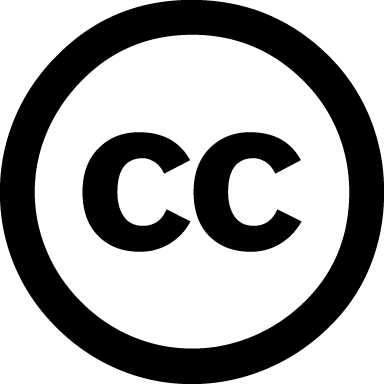https://www.dagstuhl.de/20202

# POSTPONED Geometric Logic, Constructivisation, and Automated Theorem Proving

Due to the Covid-19 pandemic, this seminar is postponed indefinitely.

## Organisatoren

Thierry Coquand (University of Gothenburg, SE)
Hajime Ishihara (JAIST – Ishikawa, JP)
Sara Negri (University of Genova, IT)
Peter M. Schuster (University of Verona, IT)

## Motivation

Right at the origin of the development of the modern concepts of proof and computation, fundamental both to mathematics and computer science, Brouwer was advocating the exclusive use of effective methods of proof, designed intuitionism, whereas Hilbert was arguing that non-effective methods are important for the progress of mathematics, viewing constructivisation as an activity to take place after the fact. While the BrouwerHilbert debate arose from epistemological concerns about mathematics, the interplay between effective and non-effective proof has become more and more relevant since the advent of theoretical and actual computing devices: We now know that, to a certain extent, intuitionistic logic can be seen as the logic of computation.

A central question has remained from that discussion: What is the extent of intuitionistic mathematics? Ordinal analysis is an attempt to describe how strong subsystems of analysis can be proved consistent in intuitionistic mathematics. Another foundational solution, with the goal of rebuilding mathematics constructively, is aimed at by Bishop-style constructive mathematics and by Martin-Löfs intuitionistic type theory, the latter most recently in the form of the so-called homotopy or univalent type theory put forward by Voevodsky.

From a more practical angle, the question rather is to which extent any given proof is effective, which proofs of which theorems can be rendered effective, and whether and how numerical information such as bounds and algorithms can be extracted from proofs. Ideally, all this is done by manipulating proofs mechanically and/or by adequate metatheorems (proof translations, automated theorem proving, program extraction from proofs, proof analysis, proof mining, etc.). In this vein, the central question should be put as follows: What is the computational content of proofs?

This Dagstuhl Seminar will be guided by this central question, putting a special focus on coherent and geometric theories and their generalisations: for they are (a) fairly widespread in mathematics and non-classical logics such as temporal and modal logics, (b) a priori amenable for constructivisation (Barrs Theorem), and (c) particularly suited as a basis for automated theorem proving. Specific topics include (i) categorical semantics for geometric theories, (ii) complexity issues of and algorithms for geometrisation of theories including speed-up questions, (iii) the use of geometric theories in constructive mathematics including finding algorithms, (iv) proof-theoretic presentation of sheaf models and higher toposes and (v) coherent logic for obtaining automatically readable proofs.

Seminar presentations of recent research and refresher tutorials of ground knowledge will be complemented by parallel work groups on the relevant topics. Tutorials of two hours each are planned about proof theory of geometric logic, sheaf models and constructive algebra, proof mining and program extraction in axiomatic environments, and automated theorem proving with coherent logic.Creative Commons BY 3.0 DEThierry Coquand, Hajime Ishihara, Sara Negri, and Peter M. Schuster

## Classification

• Data Structures / Algorithms / Complexity
• Semantics / Formal Methods
• Verification / Logic

## Keywords

• Geometric logic
• Constructivisation
• Automated theorem proving
• Proof theory
• Categorical semantics

## Dokumentation

In der Reihe Dagstuhl Reports werden alle Dagstuhl-Seminare und Dagstuhl-Perspektiven-Workshops dokumentiert. Die Organisatoren stellen zusammen mit dem Collector des Seminars einen Bericht zusammen, der die Beiträge der Autoren zusammenfasst und um eine Zusammenfassung ergänzt.

## Publikationen

Es besteht weiterhin die Möglichkeit, eine umfassende Kollektion begutachteter Arbeiten in der Reihe Dagstuhl Follow-Ups zu publizieren.

## Dagstuhl's Impact

Bitte informieren Sie uns, wenn eine Veröffentlichung ausgehend von
Ihrem Seminar entsteht. Derartige Veröffentlichungen werden von uns in der Rubrik Dagstuhl's Impact separat aufgelistet  und im Erdgeschoss der Bibliothek präsentiert.